# The MI Procedure

### Statistical Assumptions for Multiple Imputation

The MI procedure assumes that the data are from a multivariate distribution and contain missing values that can occur for any of the variables. It also assumes that the data are from a multivariate normal distribution when either the regression method or the MCMC method is used.

Supposeis the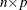matrix of complete data, which is not fully observed, and denote the observed part ofby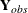and the missing part by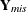. The MI and MIANALYZE procedures assume that the missing data are missing at random (MAR); that is, the probability that an observation is missing can depend on, but not on(Rubin, 1976, 1987, p. 53).

To be more precise, suppose thatis thematrix of response indicators whose elements are zero or one depending on whether the corresponding elements of Y are missing or observed. Then the MAR assumption is that the distribution ofcan depend onbut not on: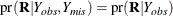For example, consider a trivariate data set with variablesand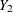fully observed, and a variablethat has missing values. MAR assumes that the probability thatis missing for an individual can be related to the individual’s values of variablesand, but not to its value of. On the other hand, if a complete case and an incomplete case forwith exactly the same values for variablesandhave systematically different values, then there exists a response bias for, and MAR is violated.

The MAR assumption is not the same as missing completely at random (MCAR), which is a special case of MAR. Under the MCAR assumption, the missing data values are a simple random sample of all data values; the missingness does not depend on the values of any variables in the data set.

Although the MAR assumption cannot be verified with the data and it can be questionable in some situations, the assumption becomes more plausible as more variables are included in the imputation model (Schafer 1997, pp. 27–28; Van Buuren, Boshuizen, and Knook 1999, p. 687).

Furthermore, the MI and MIANALYZE procedures assume that the parametersof the data model and the parameters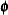of the model for the missing-data indicators are distinct. That is, knowing the values ofdoes not provide any additional information about, and vice versa. If both the MAR and distinctness assumptions are satisfied, the missing-data mechanism is said to be ignorable Rubin 1987, pp. 50–54; Schafer 1997, pp. 10–11).